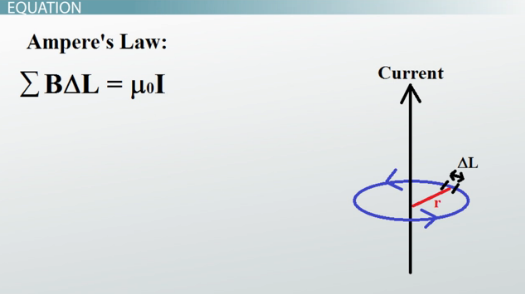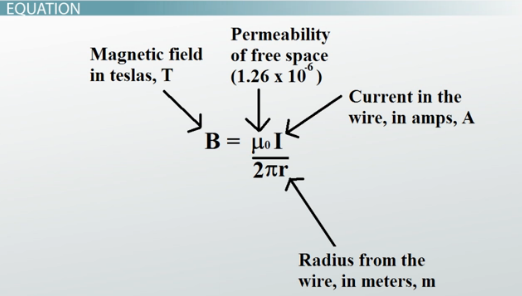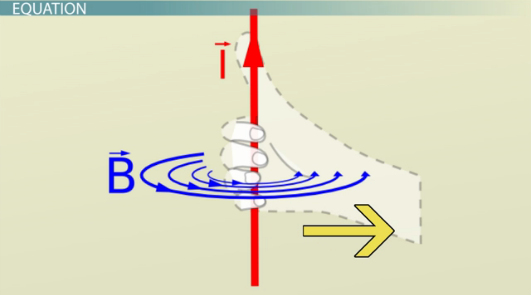# Ampere's Law: Definition & Examples

An error occurred trying to load this video.

Try refreshing the page, or contact customer support.

Coming up next: Maxwell's Equations: Definition & Application

### You're on a roll. Keep up the good work!

Replay
Your next lesson will play in 10 seconds
• 0:09 What Is Ampere's Law
• 1:08 Equation
• 3:18 Examples
• 4:33 Lesson Summary

Want to watch this again later?

Timeline
Autoplay
Autoplay
Speed

#### Recommended Lessons and Courses for You

Lesson Transcript
Instructor: David Wood

David has taught Honors Physics, AP Physics, IB Physics and general science courses. He has a Masters in Education, and a Bachelors in Physics.

After watching this video, you will be able to explain what Ampere's Law is, and use Ampere's Law for a long, straight, current-carrying wire to solve problems. A short quiz will follow.

## What Is Ampere's Law?

The full version of Ampere's Law is one of Maxwell's Equations that describe the electromagnetic force. Ampere's Law specifically says that the magnetic field created by an electric current is proportional to the size of that electric current with a constant of proportionality equal to the permeability of free space.

Stationary charges produce an electric field proportional to the magnitude of the charge. But moving charges produce magnetic fields proportional to the current (charge and movement).

The only problem with Ampere's Law is that it is a differential equation -- in other words, you need to do some calculus to use it. When you use Ampere's Law, you look at the particular situation you're in, plug in some values for that situation and complete the integral. This will get you an equation that fits the particular situation.

But instead of doing the messy calculus, we can look at the result of all that work. The situation we're going to look at in more detail is using Ampere's Law to find the magnetic field created by a long, straight, current-carrying wire.

## Equation

The field created by a long, straight, current-carrying wire is in the form of concentric circles. And as you move further from the wire, those circles get further apart -- or in other words, the field gets weaker. We could create an equation for this by using Ampere's Law and doing some calculus. But we can actually derive this equation without any calculus at all.

Instead of an integral, we'll use a sum. The sum of all the magnetic field elements that make up the concentric circle: magnetic field B times the length of the element delta-L is equal to mu-zero (permeability of free space) times the current in the wire I. This is Ampere's Law.Then realize that by summing all these elements up, your delta-L becomes the circumference of the concentric circle, 2pir. Rearrange this to make the magnetic field the subject, and you get THIS final equation for the field created by a current carrying wire.Here, B is the magnetic field at a particular point in space, measured in teslas. Mu-zero is the permeability of free space, which is a constant that's always equal to 1.257 x 10^-6. I is the current flowing through the wire, measured in amps. And r is the radial distance you are away from the wire, measured in meters. So you can use this equation to figure out the magnetic field strength at a distance r from a current-carrying wire.

Thanks to the equation, you can calculate the strength of the field. But what about the direction? For that, I need you to give me a thumbs up with your right hand. No, seriously, do it right now.

Using this diagram of a current-carrying wire, point your thumb in the direction the current is moving, in the direction of the arrow marked I. Now imagine curling your fingers around the wire, grabbing it. The direction your fingers point represents the direction the field lines point -- which way the arrows on the concentric circles go. This is called the right-hand rule, and it's vitally important you don't accidentally use your left hand, because you'll get exactly the wrong answer.## Examples

Okay, let's go through an example. Let's say you have a current-carrying wire pointing due-north. If the wire is carrying a current of 0.1 amps, what is the magnitude and direction of the magnetic field at a distance of 0.01 meters above the wire?

To unlock this lesson you must be a Study.com Member.

### Register to view this lesson

Are you a student or a teacher?

### Unlock Your Education

#### See for yourself why 30 million people use Study.com

##### Become a Study.com member and start learning now.
Back
What teachers are saying about Study.com

### Earning College Credit

Did you know… We have over 160 college courses that prepare you to earn credit by exam that is accepted by over 1,500 colleges and universities. You can test out of the first two years of college and save thousands off your degree. Anyone can earn credit-by-exam regardless of age or education level.